SPACESTATPACK: A Spatial Statistics Package in Fortran 90 1.0

(with PC executable code)

Purpose

This collection of files (Fortran 90 source code and PC executable files) implements the fast maximum likelihood computations for large numbers of observations described in:

Barry, Ronald, and R. Kelley Pace, "A Monte Carlo Estimator of the Log Determinant of Large Sparse Matrices," Linear Algebra and its Applications, Volume 289, Number 1-3, 1999, p. 41-54.

Pace, R. Kelley, and Ronald Barry, "Quick Computation of Regressions with a Spatially Autoregressive Dependent Variable," Geographical Analysis, Volume 29, Number 3, July 1997, p. 232-247.

Pace, R. Kelley, and Ronald Barry, Sparse Spatial Autoregressions, Statistics and Probability Letters, Volume 33, Number 3, May 5 1997, p. 291-297.

At the moment the package only estimates mixed regressive spatially autoregressive models of the form: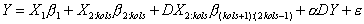where Y is the n element vector of dependent variable observations, X is the n by kols matrix of independent variable observations with the first column containing ones (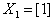), D is an n by n spatial weight matrix,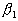is the intercept,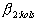is the kols-1 element parameter vector associated with the non-constant independent variables,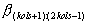is the kols-1 element parameter vector associated with the spatially lagged non-constant independent variables (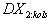), and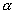is the spatial autoregressive parameter associated with the spatially lagged dependent variable (DY). This corresponds to estimating separate "spatial lags" or spatial local averages for the independent and dependent variables. It subsumes OLS with just Y and X, a SAR model with autoregressive errors (given the restriction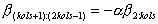), models with just spatial lags of the independent variables, and so forth.

Estimation is via maximum likelihood with the following profile or concentrated log-likelihood function,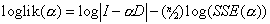where SSE(a ) is the sum-of-squared errors associated with a given value of the parameter a . As a approaches 1,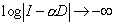. Hence, this term penalizes large values of a . Simply minimizing SSE(a ) results in upwardly biased estimates of a for spatial problems. Hence, maximum likelihood estimation is crucial for this type of spatial model.

Performance

Historically, the need to evaluate the determinant of the n by n matrix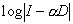has made it difficult to perform spatial estimation with many observations (large n). As of 1995, the largest spatial autoregression we could find estimated in the literature was for 2,500 observations and this required hours on a workstation or just under a minute on a supercomputer. Contrast this with Table 1 which provides a quick summary of why SPACESTATPACK exists.

 Table 1 — Performance of the Routines Across Different Datasets Harrison and Rubinfeld Data Geographical Analysis Data Statistics and Probability Letters Data number of observations (n) 506 3,107 20,640 number of neighbors (m) 4 4 4 L 20 20 20 iter 10 10 10 kols 14 5 9 Time to find Neighbors less than 1 second 3 seconds 138 seconds Time to compute estimated log-determinants less than 1 second less than 1 second 8 seconds Time needed to estimate statistical model 7 seconds 4 seconds 12 seconds Total time needed in seconds on a 233 Mhz Pentium MMX with 64 megabytes of RAM using Windows NT 4.0 9 seconds 8 seconds 158 secondsor 2.4 minutes

Hence, using different algorithms can allow PCs to substantially exceed the performance of supercomputers using brute force algorithms.

The memory requirements of SPACESTATPACK are rather modest as well. The running of the 20,640 observation spatial autoregression required under 7 megabytes of RAM for its workspace and this ran on a Pentium PC with 64 megabytes of memory.

Spatial Statistics

In spatial statistics a key element is the variance-covariance matrix which quantifies the dependence among the n observations. One variant of the literature models the inverse of this matrix, sometimes known as the concentration or precision matrix. A subset of that literature models the half-power of the precision matrix (simultaneous spatial autoregressions). To perform maximum likelihood estimation with continuous, unbounded densities, one needs to compute the log of the determinant of the precision matrix (or twice the log-determinant of the precision matrix raised to the (1/2) power).

To address this problem, Barry and Pace in the Linear Algebra and its Applications (1999) paper provide an estimator for log-determinant. They illustrate the efficacy of the estimator by using it on a 1M by 1M matrix on a PC using Matlab. This set of files implements a variant of their approach in Fortran 90.

The advantage of the Monte Carlo Log-determinant approach over matrix decompositions of n by n equations consists of (1) potentially dramatically lower memory requirements and (2) faster potential execution. The main problem with the LU or Cholesky decompositions used to the find the determinant in n by n problems are the sensitivity of these procedures to the pattern of non-zeros. The Monte Carlo procedure does not depend upon the pattern, just upon the degree of sparsity.

This approach has uses outside of statistics, but we focus on the statistical applications here.

Specifically, this particular program computeswhere I is the identity matrix and D is an n by n spatial weight matrix with non-negative entries, zeros on the main diagonal, and row-stochastic (actually this program handles only asymmetric, row-stochastic matrices while the paper describes a version for symmetric matrices). For this implementation, the m nearest neighbors to observation i have positive entries in D. For the applications to work very well for large sample sizes, most entries in D must be zero. In other words, D is sparse. For our data, anywhere from 4 to 15 neighbors has been optimal.

See the version of the Geographical Analysis article in article\GA_ms for references to standard spatial statistics works and other details.

Required Hardware and Software

The executable files run on PC compatibles running Windows 95 or Windows NT. We developed the executable files on the MS Powerstation 4 Professional version. The source code files can be compiled theoretically to any platform with a Fortran 90 compiler. However, we do use IMSL routines in the programs. Most of these could be easily replaced by other calls, but the routines used to find the nearest neighbors are more essential. This involves a quadtree data structure (4 branches instead of 2 as in binary trees) to efficiently find the neighbors.

Directories and Files Included and Required

Most users will download a zipped version of this program from the website www.spatial-statistics.com or copy this file from a CD-ROM. Extract the files to some convenient directory on your computer. If you copied files directly, these may still have the "read-only" attribute. Use the properties menu from the explorer menu to turn the "read-only" attribute off. Again, using the zip extraction program is preferred.

This parent directory includes a directory of the source code and the executable code for win/95,win-nt. In addition, it has subdirectories for data and articles.

In the execute subdirectory, the file nnweight1.exe computes the m nearest neighbors used in constructing the spatial weight matrix D, the file moments1.exe computes moments used in the computation of the log-determinant, the file logdet1.exe actually computes the log-determinant over a grid of 100 values of a , the autoregressive parameter which lies between 0 and 1 (we restrict our attention to this interval based on subject matter considerations), and the file fmix1.exe computes the estimates using the mixed regressive spatially autoregressive model.

In the execute subdirectory, to use these programs to compute the log-determinant, run the batch file, mcdet.bat by double-clicking on it. If you set the parameters on your command windows appropriately in Windows NT, you can scroll through the output, cut and paste, etc. In Windows NT you can also redirect the screen output to a file by running mcdet.bat>filename. In Window 95 the command window does not work as well (nor do the redirection features) and so the best way of obtaining output is to redirect the output to a series of files corresponding to the individual executable files. The supplied batch file, verbosely named Mcdet_win95_redirect.bat saves the output of nnweight1.exe to neighbor_out1.txt, moment1.exe to moments_out1.txt, logdet1.exe to logdet_out1.txt, and finally fmix1.exe save its output to stat_out1.txt. This last file, stat_out1.txt is the one containing the actual statistical analysis output. One can open these files using the program "Notepad" supplied with windows or a word processing program. Try double-clicking on the file. Most of the time this will allow you to view it. Window NT users can also double-click on the file Mcdet_win95_redirect.bat to produce output in the various text files.

You must provide the input files xcoord.asc, ycoord.asc, mcparms.asc, wvec.asc, xdata.asc, and ydata.asc.

The ascii files xcoord.asc, ycoord.asc contain the x,y locational points on the plane for the n observations (e.g., East-West, North-South coordinates). These are n element vectors.

The file xdata.asc has the independent variables in row order (each row in the file corresponds to an observation). The first column should be ones (the intercept variable). The remaining variables should be non-constant. Like most regression software, the program prefers reasonable scaling and a data matrix with full rank.

The file ydata.asc contains the n dependent variable observations.

The file mcparms.asc contains 6 parameters. Specifically, it contains:

[n mold m L iter kols]

For example,

3107 10 4 100 50 5

means 3107 observations (n), a maximum of 10 neighbors searched for (mold), 4 actually used in the construction of the moments and the estimates (m), 100 moments computed (L), with 50 iterations (iter), and 5 independent variables examined (kols). The second element or number (mold) should be at least as high as the third element or number (m). The number of independent variables (kols) includes one intercept and the rest of the variables are non-constant. The accuracy of the log-determinant approximation increases with the number of moments L (probably should never need more than 100) and with the number of iterations iter (10 is a good starting point). If the range of the optimal a varies greatly from using the lower confidence bound of the estimated log-determinant to the upper confidence bound of the log-determinant, one should increase L or iter.

The file wvec.asc contains the weighting used among neighbors (e.g. .25 .25 .25 .25 for 4 neighbors). This allows one to make the nearest neighbor more important than the next nearest neighbor, etc. Each of these numbers must be non-negative and collectively they must sum to 1.

The output appears in nnmat.asc, nndis.asc, ucuasc.asc, detrez.asc, detrezfor.asc, loglikmat.asc, loglikvec0.asc, alphamat.asc, bests.asc, and hypothesis_matrix.asc.

The file nnmat.asc contains the indices of the mold neighbors to observation i.

The file nndis.asc contains the Euclidean distances from the observation to the nearest neighbors.

The file ucuasc.asc contains all the estimated moments used in the construction of the estimated log-determinant.

The file detrez.asc contains the autoregressive parameter, lower confidence (95%) bound on log-determinant, estimated log-determinant, and upper bound of the log-determinant by row.

The file detrezfor.asc contains the same information as in detrez.asc but is organized in column order (which Fortran prefers) for use in fmix1.exe.

The file loglikmat.asc contains the maximum likelihoods by model (rows). By column it gives the maximum of the log-likelihood using the lower bound of the log-determinant, the maximum of the log-likelihood using the estimated log-determinant, and the maximum of the log-likelihood using the upper bound of the log-determinant.

The file loglikvec0.asc saves the log-likelihoods associated with alpha=0 by model.

The file alphamat.asc contains the ML alphas by model (rows). By column it gives the optimal a using the lower bound of the log-determinant, the optimal a for the estimated log-determinant, and the optimal a using the upper bound of the log-determinant.

The file bests.asc contains the model number,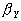from OLS on Y,from OLS on DY, and.

The file hypothesis_matrix.asc gives the variables included (by row) versus the model (by column).

The screen output displays more information than saved on disk. We recommend logging and/or redirecting the output.

One User’s Suggestions

David Brasington, Department of Economics, Tulane University provides the following suggestions for quickly getting started.

a. Make infiles xcoord.asc, ycoord.asc, mcparms.asc, wvec.asc, xdata.asc, and ydata.asc.

b. Delete the infiles currently in Execute folder.

c. Copy your infiles to Execute folder.

d. Double-click mcdet_win95_redirect.bat (or however you do non-Windows one).

e. Do not close the execute window, but open your output files now (especially stat_out1.txt) in a text editor or word processing program to view them.

f. Save your outfiles in your text editor or word processing program under different names if you want to save your output.

Included Examples

We have included two example datasets with parameter files to aid in getting started with the package. These appear in their own directories. In addition, the package comes with the Geographical Analysis example in the execute subdirectory and hence all one needs to run it is to double-click on the file mcdet.bat or the file Mcdet_win95_redirect.bat and view the text files it produces.

Geographical Analysis Example

As our first example, we replicate the empirical work in the Pace and Barry (1997) Geographical Analysis article which studied spatial statistical aspects of voter participation across the US. A pdf version of the article resides in articles\GA_ms (we have copyright permission from Ohio State University).

The various data and parameter files appear in the subdirectory data\gadata. Specifically, the file xdata.asc contains the independent data variables and the file ydata.asc contains the dependent variable data. The files ycoord.asc and xcoord.asc contain the x and y spatial coordinates. We have included an example of a typical run which appears in the file typrun.txt. The file mcparms.asc contains the relevant parameters to make the routine work (n=3107, mold=8, m=4, L=100, iter=10, kols=5). Having the parameter mold>m, allows one to increase m in computing the log-determinants and the statistical estimation of the model without having to recompute all the nearest neighbors. This facilitates the optimization of the log-likelihood over the parameter m. In the article, the choice of m was arbitrary and set to 4 neighbors. There are 3107 observations and 5 variables in the non-spatial model (including intercept). In computing the Monte Carlo estimate of the log-determinant, we set L, the number of moments considered, to 100 (one should not need more than this for almost all problems — this example would run fine with a smaller number). We set iter to 10, a relatively small number, but one which proves sufficient for obtaining enough accuracy for the maximum likelihood computations. Decreased values of L and iter lead to shorter execution times at the expense of possible accuracy. We will discuss later how to gauge this trade-off.

In this article, we used data on the total number of votes cast in the 1980 presidential election per county (Votes), the population in each county of 18 years of age or older (Pop), the population in each county with a 12th grade or higher education (Education), the number of owner-occupied housing units (Houses), and the aggregate income (Income). The x-coordinates and y-coordinates came from 3107 geographic centroids of selected counties in the US. The original latitude and longitude data have been projected.

We elected to examine the log of the proportion of votes cast for both candidates in the 1980 presidential election. Hence, we can express our dependent variable as ln(PrVotes) = ln(Votes/ Pop) = ln(Votes) – ln(Pop). We fitted the following model via OLS (which uses kols variables):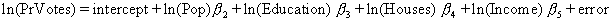The corresponding mixed regressive spatially autoregressive model is,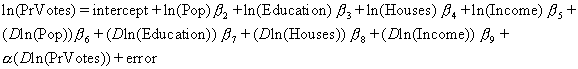where the first line contains the same variables as the first model, the second line contains the spatially lagged (or locally averaged) independent variables, and the third line contains the spatially lagged dependent variable. The row-stochastic (row weights sum to 1) matrix D acts much like a lag operator does in time series. This matrix takes an average of the relevant variable’s values for the neighboring observations (defined by the m nearest neighbors). One can think of D as a linear filter, as a nonparametric estimator (nearest neighbor smoother) of local aspects of the data, or as an identifier of a the other members of the cluster containing observation i (nearest neighbor computations are extensively used in cluster analysis – a difference here is the membership of an observation to multiple clusters). This model subsumes or nests both OLS, a model where spatially lagged independent variables matter, a model where the spatially lagged dependent variable matters, and a spatial autoregression in the errors. Hence, the mixed regressive spatially autoregressive model is quite general and a good initial model to run. If the model does not substantially improve upon OLS in sample errors, this indicates the spatial effects probably do not critically matter.

To assess this, examine the "summary of estimates" section of the output. The estimated log-likelihood for the unrestricted model (ML on model 1) is –5680.0 (with a lower 95% confidence bound of –5685.2 and a upper 95% confidence bound of –5674.6). In contrast, OLS on the non-spatial variables (Model 2 with a =0) has a log-likelihood of –6307.1. The unrestricted Model 1 with estimated a uses 10 parameters while OLS on Model 2 with a =0 uses 5 parameters. Hence, under the null hypothesis of no difference, twice the likelihood ratio should follow a chi-squared density with 5 degrees-of-freedom. Obviously, one would reject the null hypothesis in this case.

 Table 2 — Likelihood Ratio Tests Verbal Description Mathematical Description Relevant Output Example Unrestricted ML on mixed regressive spatially autoregressive model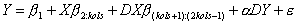Model 1 with estimated a -5680.0 OLS on mixed regressive model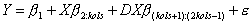Model 1 with a =0 -6229.9 ML on spatially autoregressive model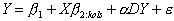Model 2 with estimated a -5868.1 OLS on non-spatial model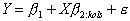Model 2 with a =0 -6307.1 Deletion of intercept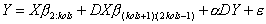Model 3 with estimated a -5726.8 Deletion of first independent variable and its spatial lagModel 4 with estimated a -6121.5 Simultaneous Autoregressive Errors (SAR) model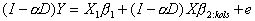(corresponds to the restriction) Not currently present but an important subset of the mixed regressive spatially autoregressive model

The hypothesis matrix provides a detailed description of which independent variables enter into which model and is essential for understanding the correspondence between the Model number and the statistical model estimated. This appears early in the "Summary of Estimates" section.

The output summarizing the optimal or ML a s provides some insights. First, high variation relative to subject matter considerations across columns for a particular row indicate the need to increase L or iter. Note, even with as few as 10 iterations, the log-determinant estimation was precise enough to cause little variation in the estimated autoregressive parameter, a . Using the lower confidence bound of the log-determinant in the log-likelihood resulted in an optimal a of 0.61, while using the upper confidence bound resulted in a of 0.62. This small lack of precision in the estimated parameter should cause relatively few problems in most applications. As an additional check, the exact optimal log-likelihood was -5679.09 and the estimated optimal log-likelihood was -5680.0.

If the optimal a from using the lower and upper confidence intervals diverges too much but appears approximately centered around the optimal a associated with the estimated log-determinant, one should increase iter. If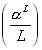is not close to zero, increase L.

\$\$\$\$\$\$\$\$\$\$\$\$\$\$\$\$\$\$\$\$\$ SUMMARY OF ESTIMATES \$\$\$\$\$\$\$\$\$\$\$\$\$\$\$\$\$\$\$\$\$\$\$\$\$\$\$

Overall Summary of Max Log-Liks, Optimal Alpha by Model

To put everything in one place, we repeat the display of the hypothesis

matrix and its interpretation

The following matrix details the sequence of estimated models.

The columns (labeled 1,2,... in the first row) vary across the models.

The rows show the variables included for each model.

The first model is the unrestricted model,

the second model uses only the non-spatial independent variables,

the third model is without intercept,

and subsequent models correspond to deleting a variable and

its spatial lag from the unrestricted model.

hypothesis matrix

1 2 3 4 5 6 7

1 1 1 0 1 1 1 1

2 1 1 1 0 1 1 1

3 1 1 1 1 0 1 1

4 1 1 1 1 1 0 1

5 1 1 1 1 1 1 0

6 1 0 1 0 1 1 1

7 1 0 1 1 0 1 1

8 1 0 1 1 1 0 1

9 1 0 1 1 1 1 0

Optimal Log-liks by model (row) for lower con.,average,upper con. log-dets

Optimal logliks by model

1 2 3

1 -5685.2 -5680.0 -5674.6

2 -5871.2 -5868.1 -5865.1

3 -5733.3 -5726.8 -5720.2

4 -6126.9 -6121.5 -6116.1

5 -5819.4 -5811.2 -5802.8

6 -6050.5 -6045.9 -6041.3

7 -5699.1 -5693.6 -5687.9

Optimal alphas by model (row) for lower con.,average,upper con. log-dets

Optimal Alphas

1 2 3

1 .6100 .6100 .6200

2 .4900 .4900 .4900

3 .6600 .6600 .6700

4 .6100 .6200 .6200

5 .7100 .7200 .7200

6 .5700 .5800 .5900

7 .6200 .6200 .6300

log-liks by model for alpha=0

1 -6229.9

2 -6307.1

3 -6434.2

4 -6692.7

5 -6759.9

6 -6508.8

7 -6280.4

Note, the first element of the log-likelihoods for alpha=0 vector

corresponds to the model of Y regressed on X and spatially lagged X.

The second element corresponds to the model of Y regressed on X.

The third element corresponds to the model sans intercept

Subsequent elements correspond to the model of Y regressed on X sans

one of the independent variables and its spatial lag.

From the matrix of optimal log-likelihoods and the vector of log-likelihoods

given alpha=0, one can easily construct likelihood ratio statistics for

most of the standard hypotheses:

Spatial ML vs OLS using just Y,X, For example:

LR=max likelihood from model 1 - log-likelihood given alpha=0 for model 2

Spatial ML vs OLS using Y, X and spatially lagged X, For example:

LR=max likelihood from model 1 - log-likelihood given alpha=0 for model 1

Overall Spatial ML vs Spatial ML using X,Y,spatially lagged Y (no spatial X)

LR=max likelihood from model 1 - max likelihood from model 2

Overall Spatial ML vs Spatial ML for sub-models, etc. For example:

LR=max likelihood from model 1 - max likelihood from model (4,...)

\$\$\$\$\$\$\$\$\$\$\$\$\$\$\$\$\$\$\$\$\$\$\$\$ END OF SUMMARY \$\$\$\$\$\$\$\$\$\$\$\$\$\$\$\$\$\$\$\$\$\$\$\$\$\$\$\$\$\$\$

For each model, the program estimates it for 100 different values of the autoregressive parameter a . The use of the log-determinants across a grid of the autoregressive parameter values greatly accelerates the maximum likelihood computations involved in the estimation. The grid of values for a include 0 and the most common hypotheses involve a =0 or a =ML a . However, the entire set of values defines the profile likelihood parameterized by a . The profile likelihood values appear in the individual model estimation sections in the output. The profile likelihoods also allow construction of confidence intervals for a .

Profile log-likelihood in Alpha

1 2 3

1 -6229.9 -6229.9 -6229.9

2 -6215.2 -6215.2 -6215.2

3 -6200.7 -6200.7 -6200.6

4 -6186.2 -6186.2 -6186.2

5 -6171.9 -6171.9 -6171.9

6 -6157.7 -6157.7 -6157.7

7 -6143.6 -6143.6 -6143.6

8 -6129.7 -6129.6 -6129.6

9 -6115.9 -6115.8 -6115.7

10 -6102.2 -6102.1 -6102.0

11 -6088.6 -6088.5 -6088.4

12 -6075.2 -6075.1 -6075.0

13 -6061.9 -6061.8 -6061.6

14 -6048.8 -6048.6 -6048.5

15 -6035.8 -6035.6 -6035.4

16 -6023.0 -6022.8 -6022.6

17 -6010.3 -6010.1 -6009.8

18 -5997.8 -5997.6 -5997.3

19 -5985.5 -5985.2 -5984.9

20 -5973.4 -5973.0 -5972.6

21 -5961.4 -5961.0 -5960.6

22 -5949.6 -5949.1 -5948.7

23 -5937.9 -5937.4 -5936.9

24 -5926.5 -5926.0 -5925.4

25 -5915.3 -5914.7 -5914.1

26 -5904.2 -5903.6 -5902.9

27 -5893.4 -5892.7 -5892.0

28 -5882.7 -5882.0 -5881.2

29 -5872.3 -5871.5 -5870.7

30 -5862.1 -5861.2 -5860.3

31 -5852.1 -5851.2 -5850.2

32 -5842.4 -5841.3 -5840.3

33 -5832.9 -5831.8 -5830.6

34 -5823.6 -5822.4 -5821.2

35 -5814.6 -5813.3 -5812.0

36 -5805.8 -5804.4 -5803.0

37 -5797.2 -5795.8 -5794.3

38 -5789.0 -5787.4 -5785.9

39 -5781.0 -5779.3 -5777.7

40 -5773.2 -5771.5 -5769.7

41 -5765.8 -5763.9 -5762.1

42 -5758.6 -5756.7 -5754.7

43 -5751.7 -5749.7 -5747.6

44 -5745.2 -5743.0 -5740.8

45 -5738.9 -5736.6 -5734.3

46 -5732.9 -5730.5 -5728.0

47 -5727.3 -5724.7 -5722.1

48 -5722.0 -5719.2 -5716.5

49 -5717.0 -5714.1 -5711.2

50 -5712.3 -5709.3 -5706.3

51 -5708.0 -5704.8 -5701.7

52 -5704.0 -5700.7 -5697.4

53 -5700.4 -5696.9 -5693.4

54 -5697.2 -5693.5 -5689.9

55 -5694.3 -5690.5 -5686.6

56 -5691.8 -5687.8 -5683.8

57 -5689.7 -5685.5 -5681.3

58 -5688.0 -5683.6 -5679.2

59 -5686.7 -5682.1 -5677.5

60 -5685.8 -5681.0 -5676.1

61 -5685.3 -5680.2 -5675.2

62 -5685.2 -5680.0 -5674.7

63 -5685.6 -5680.1 -5674.6

64 -5686.4 -5680.7 -5675.0

65 -5687.7 -5681.7 -5675.7

66 -5689.4 -5683.2 -5677.0

67 -5691.6 -5685.1 -5678.6

68 -5694.3 -5687.5 -5680.7

69 -5697.5 -5690.4 -5683.3

70 -5701.2 -5693.8 -5686.4

71 -5705.4 -5697.7 -5690.0

72 -5710.1 -5702.1 -5694.1

73 -5715.4 -5707.0 -5698.7

74 -5721.2 -5712.5 -5703.8

75 -5727.6 -5718.5 -5709.4

76 -5734.6 -5725.1 -5715.6

77 -5742.2 -5732.3 -5722.4

78 -5750.4 -5740.1 -5729.8

79 -5759.2 -5748.4 -5737.7

80 -5768.7 -5757.5 -5746.3

81 -5778.9 -5767.2 -5755.5

82 -5789.7 -5777.5 -5765.3

83 -5801.4 -5788.6 -5775.9

84 -5813.8 -5800.5 -5787.2

85 -5827.0 -5813.1 -5799.2

86 -5841.0 -5826.5 -5812.0

87 -5856.0 -5840.8 -5825.6

88 -5871.9 -5856.0 -5840.1

89 -5888.8 -5872.1 -5855.5

90 -5906.8 -5889.3 -5871.9

91 -5926.0 -5907.7 -5889.4

92 -5946.6 -5927.3 -5908.0

93 -5968.7 -5948.3 -5928.0

94 -5992.5 -5970.8 -5949.3

95 -6018.8 -5995.1 -5972.4

96 -6049.2 -6021.6 -5997.4

97 -6088.8 -6050.6 -6024.8

98 -6157.9 -6082.9 -6055.2

99 -6349.7 -6119.7 -6089.5

100 -7310.7 -6162.7 -6129.5

Also, the estimates for the usual parameters appear in this section. The first column corresponds to running OLS on the dependent variable without the dependent variable spatial lag (a =0). The second column corresponds to running OLS on the dependent variable DY without the dependent variable spatial lag (a =0). The third column gives the mixed regressive spatially autoregressive ML estimates.

b from Y,b from DY, b ML

1 2 3

1 1.167 1.153 .464

2 -.729 -.019 -.718

3 .223 .052 .191

4 .452 .002 .451

5 .007 -.032 .026

6 -.069 -.762 .396

7 .399 .566 .054

8 -.061 .372 -.289

9 -.258 -.216 -.126

See the Geographical Analysis article for more detail on the interpretation of these estimates.

Harrison and Rubinfeld Example

Harrison and Rubinfeld (1978) investigated various methodological issues related to the use of housing data to estimate the demand for clean air. The specific reference to this is Harrison, David, and Daniel L. Rubinfeld, "Hedonic Housing Prices and the Demand for Clean Air," Journal of Environmental Economics and Management, Volume 5, (1978), 81-102. They illustrated their procedures using 506 observations on housing from the Boston SMSA. This has been a well-analyzed data set. See the data\hrdata subdirectory for more information. The specific dataset used here is the corrected one discussed in Gilley and Pace (1996). This specific version is in mean-differenced form (except for the intercept). The reference to this is Gilley, O.W., and R. Kelley Pace, "On the Harrison and Rubinfeld Data," Journal of Environmental Economics and Management, 31 (1996), 403-405. A pdf version of the article appears in the subdirectory articles\HR_ms (we have copyright permission from Academic Press). Pace and Gilley (1997) added x and y coordinates (e.g., EW, NS coordinates) to the data and estimated a SAR model. The reference to this is Pace, R. Kelley, and O.W. Gilley, "Using the Spatial Configuration of the Data to Improve Estimation," Journal of the Real Estate Finance and Economics 14 (1997), 333-340. The mixed regressive spatially autoregressive model estimated here significantly improves on SAR (which it subsumes) for this example.

In the subdirectory data\hrdata the files xdata.asc contain the independent data variables and the files ydata.asc contain the dependent variable data. The files ycoord.asc and xcoord.asc contain the latitude and longitude variables. The file mcparms.asc contains the relevant parameters to make the routine work.

Modeling Strategies to Minimize Computational Costs

Some operations cost little and other operations cost more in changing the overall estimation problem. For example, changing the definition of X, Y costs very little. This just means one need rerun fmix1.exe, a relatively quick operation. Hence, one can quickly examine a number of possible models, transformations, and reweightings (with easy side accounting of the effect on the log-likelihood). Most researchers seem to spend much of their time in this stage of estimation.

Changing the number of neighbors m (as long as m is not greater than mold) also costs less than rerunning all the programs as mold of these have already been stored by the nearest neighbor program, nnweight1.exe. Changing m or wvec, the weighting of the neighbors, requires recomputation of the log-determinant using the programs moments1.exe and logdet1.exe. Naturally, this also means recomputing the estimates by running fmix1.exe.

Finally, changing the number of observations is the most expensive operation. In this case all of the programs need to be rerun.

We suggest you run the program initially with L equal to 20 and iter set to 10. Ifusing the estimated a is more than 0.01 (this is just an approximate level), increase L. If the a obtained from using the lower confidence bound of the log-determinant in the log-likelihood differs greatly from the a obtained from using the upper confidence bound of the log-determinant of the log-likelihood, increase iter. Hence, one can begin with some approximate yet inexpensive computations and iterate to the desired degree of accuracy. Note, these steps do not require recomputation of the neighbors.

Conclusion and Future Plans

Spatial statistics has historically raised numerical difficulties. We believe SPACESTATPACK, albeit limited in scope, should make maximum likelihood estimation on large problems practical (or repeated application on smaller problems such as one might encounter in resampling). Currently, we plan to add SAR estimation and a front end for Matlab and Gauss users to access SPACESTATPACK on the PC platform. We also plan to add other metrics for picking nearest neighbors.

We encourage anyone to use our software. However, we request you cite our work. We solicit information about your experiences with the software. Thank you.

Acknowledgements

I would like to thank David Brasington, Otis Gilley, Carlos Slawson, Robby Singh, Rui Li, and Jennifer Loftin for their testing or other help with SPACESTATPACK. We would also like to thank the University of Alaska and Louisiana State University for their research support. In particular, we would like to acknowledge specific support from the LSU Real Estate Research Institute and from the Center for Real Estate at the University of Connecticut.

Contact Information

Kelley Pace

LREC Endowed Chair of Real Estate

2164 CEBA, Department of Finance

Louisiana State University

Baton Rouge, LA 70803-6308

Ron Barry

Department of Mathematics